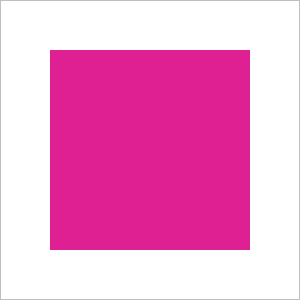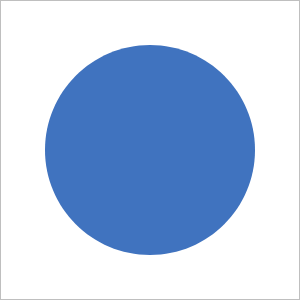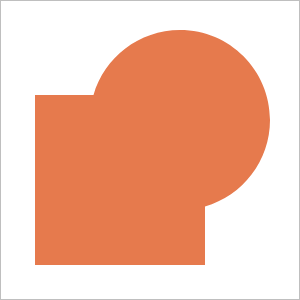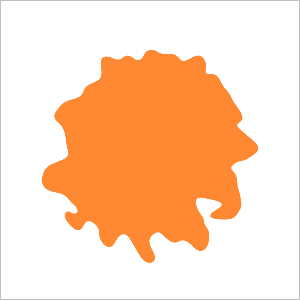# Shapes

```@renderable((300, 300))
def rectangle(r):
return P().rect(r.inset(50)).f(hsl(0.9, 0.75))
```That’s how to draw a rectangle with a 50px padding around the edges (the padding comes from the `r.inset(50)` call).

I’m re-reading this now and if you’re thinking to yourself: that’s a square — well that makes sense, but a square’s just a rectangle with the same width & height.

```@renderable((300, 300))
def oval(r):
return P().oval(r.inset(45)).f(hsl(0.6))
```That’s an oval. Sweeet.

What if you want to combine an oval and a rect?

```@renderable((300, 300))
def ovalrect(r):
return (P()
.oval(r.inset(60))
.translate(30, 30)
.union(P()
.rect(r.inset(65))
.translate(-30, -30))
.f(hsl(0.05, l=0.6, s=0.75)))
```Or maybe you want just the parts of those two shapes that don’t overlap? And maybe you want to fill the shape with a gradient and rotate the rect a little bit and then eyeball how it should be centered in its frame?

```@renderable((300, 300))
def ovalrect_diff(r):
return (P()
.oval(r.inset(60))
.translate(30, 30)
.xor(P()
.rect(r.inset(65))
.translate(-30, -30)
.rotate(-5))
hsl(0.05, l=0.6, s=0.75),
hsl(0.8, l=0.6, s=0.5)))
.translate(7)) # & eyeball it
```## Modifying Shapes

Here’s an example of building up a chain of effects to modify a simple vector shape.

```from coldtype.fx.skia import phototype

@renderable((300, 300))
def ovalmod(r):
return (P()
.oval(r.inset(60))
.flatten(5)
# breaks the oval down into non-curves, 5 is the length of the segment
.roughen(50)
# randomizes the vertices of the shape
.f(1)
.ch(phototype(r, blur=5, cut=100, cutw=5, fill=hsl(0.07, 1, 0.6))))
```